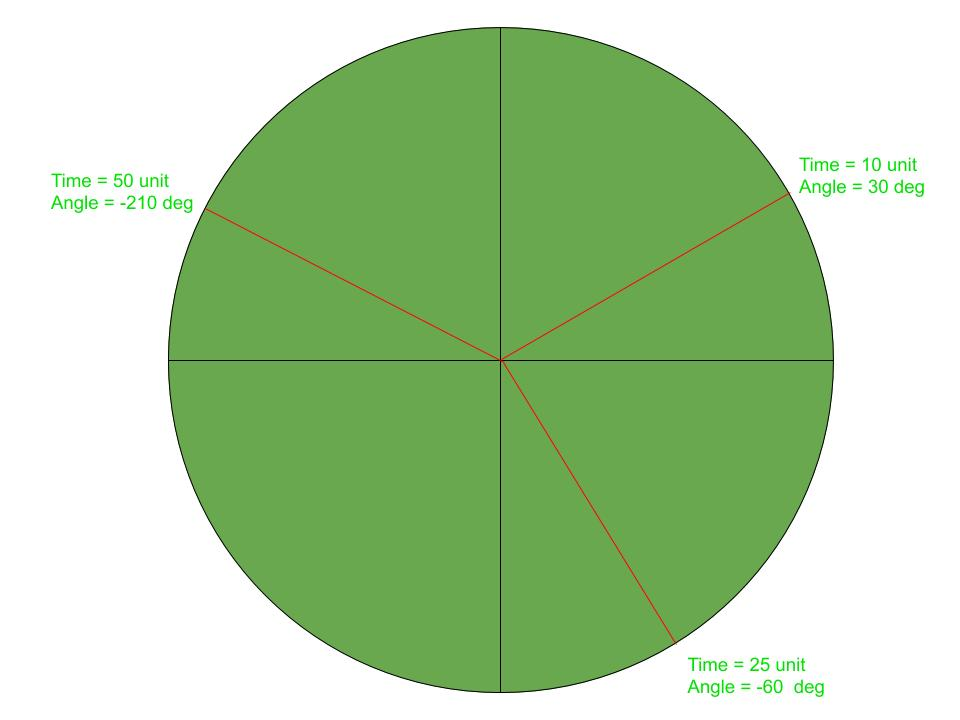# Java Applet | How to display an Analog Clock

In this article, we shall be animating the applet window to show an Analog Clock with a 1-second delay. The idea is to display the system time of every instance.Approach:
Each hand of the clock will be animating with 1-second delay keeping one end at the centre. The position of the other end can be derived by the system time. The angle formed by a hand of the clock in every second will be different throughout its journey. This is why various instances make a different angle to the horizontal line.

How to run:

```1. Save the file as analogClock.java
and run the following commands.
2. javac analogClock.java
3. appletviewer analogClock.java
```

Below is the implementation of the above approach:

Program:

 `// Java program to illustrate ` `// analog clock using Applets ` ` `  `import` `java.applet.Applet; ` `import` `java.awt.*; ` `import` `java.util.*; ` ` `  `public` `class` `analogClock ``extends` `Applet { ` ` `  `    ``@Override` `    ``public` `void` `init() ` `    ``{ ` `        ``// Applet window size & color ` `        ``this``.setSize(``new` `Dimension(``800``, ``400``)); ` `        ``setBackground(``new` `Color(``50``, ``50``, ``50``)); ` `        ``new` `Thread() { ` `            ``@Override` `            ``public` `void` `run() ` `            ``{ ` `                ``while` `(``true``) { ` `                    ``repaint(); ` `                    ``delayAnimation(); ` `                ``} ` `            ``} ` `        ``}.start(); ` `    ``} ` ` `  `    ``// Animating the applet ` `    ``private` `void` `delayAnimation() ` `    ``{ ` `        ``try` `{ ` ` `  `            ``// Animation delay is 1000 milliseconds ` `            ``Thread.sleep(``1000``); ` `        ``} ` `        ``catch` `(InterruptedException e) { ` `            ``e.printStackTrace(); ` `        ``} ` `    ``} ` ` `  `    ``// Paint the applet ` `    ``@Override` `    ``public` `void` `paint(Graphics g) ` `    ``{ ` `        ``// Get the system time ` `        ``Calendar time = Calendar.getInstance(); ` ` `  `        ``int` `hour = time.get(Calendar.HOUR_OF_DAY); ` `        ``int` `minute = time.get(Calendar.MINUTE); ` `        ``int` `second = time.get(Calendar.SECOND); ` ` `  `        ``// 12 hour format ` `        ``if` `(hour > ``12``) { ` `            ``hour -= ``12``; ` `        ``} ` ` `  `        ``// Draw clock body center at (400, 200) ` `        ``g.setColor(Color.white); ` `        ``g.fillOval(``300``, ``100``, ``200``, ``200``); ` ` `  `        ``// Labeling ` `        ``g.setColor(Color.black); ` `        ``g.drawString(``"12"``, ``390``, ``120``); ` `        ``g.drawString(``"9"``, ``310``, ``200``); ` `        ``g.drawString(``"6"``, ``400``, ``290``); ` `        ``g.drawString(``"3"``, ``480``, ``200``); ` ` `  `        ``// Declaring variables to be used ` `        ``double` `angle; ` `        ``int` `x, y; ` ` `  `        ``// Second hand's angle in Radian ` `        ``angle = Math.toRadians((``15` `- second) * ``6``); ` ` `  `        ``// Position of the second hand ` `        ``// with length 100 unit ` `        ``x = (``int``)(Math.cos(angle) * ``100``); ` `        ``y = (``int``)(Math.sin(angle) * ``100``); ` ` `  `        ``// Red color second hand ` `        ``g.setColor(Color.red); ` `        ``g.drawLine(``400``, ``200``, ``400` `+ x, ``200` `- y); ` ` `  `        ``// Minute hand's angle in Radian ` `        ``angle = Math.toRadians((``15` `- minute) * ``6``); ` ` `  `        ``// Position of the minute hand ` `        ``// with length 80 unit ` `        ``x = (``int``)(Math.cos(angle) * ``80``); ` `        ``y = (``int``)(Math.sin(angle) * ``80``); ` ` `  `        ``// blue color Minute hand ` `        ``g.setColor(Color.blue); ` `        ``g.drawLine(``400``, ``200``, ``400` `+ x, ``200` `- y); ` ` `  `        ``// Hour hand's angle in Radian ` `        ``angle = Math.toRadians((``15` `- (hour * ``5``)) * ``6``); ` ` `  `        ``// Position of the hour hand ` `        ``// with length 50 unit ` `        ``x = (``int``)(Math.cos(angle) * ``50``); ` `        ``y = (``int``)(Math.sin(angle) * ``50``); ` ` `  `        ``// Black color hour hand ` `        ``g.setColor(Color.black); ` `        ``g.drawLine(``400``, ``200``, ``400` `+ x, ``200` `- y); ` `    ``} ` `} `

Output:

Attention reader! Don’t stop learning now. Get hold of all the important Java and Collections concepts with the Fundamentals of Java and Java Collections Course at a student-friendly price and become industry ready.

My Personal Notes arrow_drop_upCheck out this Author's contributed articles.

If you like GeeksforGeeks and would like to contribute, you can also write an article using contribute.geeksforgeeks.org or mail your article to contribute@geeksforgeeks.org. See your article appearing on the GeeksforGeeks main page and help other Geeks.

Please Improve this article if you find anything incorrect by clicking on the "Improve Article" button below.

Article Tags :
Practice Tags :

1

Please write to us at contribute@geeksforgeeks.org to report any issue with the above content.